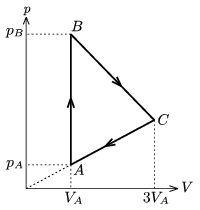Mathematical and Physical Journal
for High Schools
Issued by the MATFUND Foundation
 Already signed up? New to KöMaL?

#Problem P. 3869. (February 2006)

P. 3869. A sample of ideal gas is taken through the cyclic process shown in the figure. The temperature of the gas at state A is TA=200 K. At states B and C the temperature of the gas is the same.a) What is the greatest temperature of the gas during the cyclic process?

b) Graph the cyclic process in the T-V diagram.

(4 pont)

Deadline expired on March 13, 2006.

### Statistics:

 125 students sent a solution. 4 points: 54 students. 3 points: 30 students. 2 points: 2 students. 1 point: 22 students. 0 point: 8 students. Unfair, not evaluated: 9 solutionss.

Problems in Physics of KöMaL, February 2006